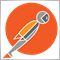# Check Code210

i just wanted to check this example : //input in EA, takeprofit =30; stoploss =0;  //void akeprofitmodify(); if(Ask< TakeProfit) OrderModify(OrderTicket(),Ask,0,Ask+30*Point,0,0);
Is this correct? Thank you.30

Is your program converting the takeprofit into a double?210

dingo34:
Is your program converting the takeprofit into a double?

its assumued that tp=30 pips being converted to a double.130282

MThomp:
i just wanted to check this example : //input in EA, takeprofit =30; stoploss =0;  //void akeprofitmodify(); if(Ask< TakeProfit) OrderModify(OrderTicket(),Ask,0,Ask+30*Point,0,0);
Is this correct? Thank you.
It is not correct - you are comparing incompatible values210

It is not correct - you are comparing incompatible values
``` just wanted to check this example : //input in EA, Takeprofit =30; stoploss =0;  //void Takeprofitmodify(); if(TakeProfit>0) OrderModify(OrderTicket(),Ask,0,Ask+(30*Point,0,clrNONE);
Is this correct? Thank you.```2388

There are so many fundamental flaws, it's time to stop and think about what you want to do.

• This is a (implicit) Long position
• Longs close @ the Bid not the Ask
• The price will always be less than the TP so even if the code were right (it's not, like Mladen says), the logic would ensure that all it does is constantly move the TP within the specified points of whatever the current price is, therefore it serves no purpose.210

nicholi shen:

There are so many fundamental flaws, it's time to stop and think about what you want to do.

• This is a (implicit) Long position
• Longs close @ the Bid not the Ask
• The price will always be less than the TP so even if the code were right (it's not, like Mladen says), the logic would ensure that all it does is constantly move the TP within the specified points of whatever the current price is, therefore it serves no purpose.

The correct term or format for  'OrderModify'  to be written where  for example ,TP=80, distance=30. (assuming TP is currently zero).